Ben has 9 ladybugs and 5 crickets in his jar. Jill has 8 ladybugs and 7 crickets in her jar. Dad thinks they have the same amount of insects in each jar. Is Dad correct? Show and tell how you know.

Assessment

## Plan

#### Formal Mathematical Language and Symbolic Notation

A student may independently select a printed number line, number chart, ten frames, graph paper, etc. as they work on a task.

### Suggested materials

Engagement Image:

Teachers may project the images below to launch this task for their students, define nouns, promote discussion, access prior knowledge, and inspire engagement and problem solving.

## Solutions tabs

### Expert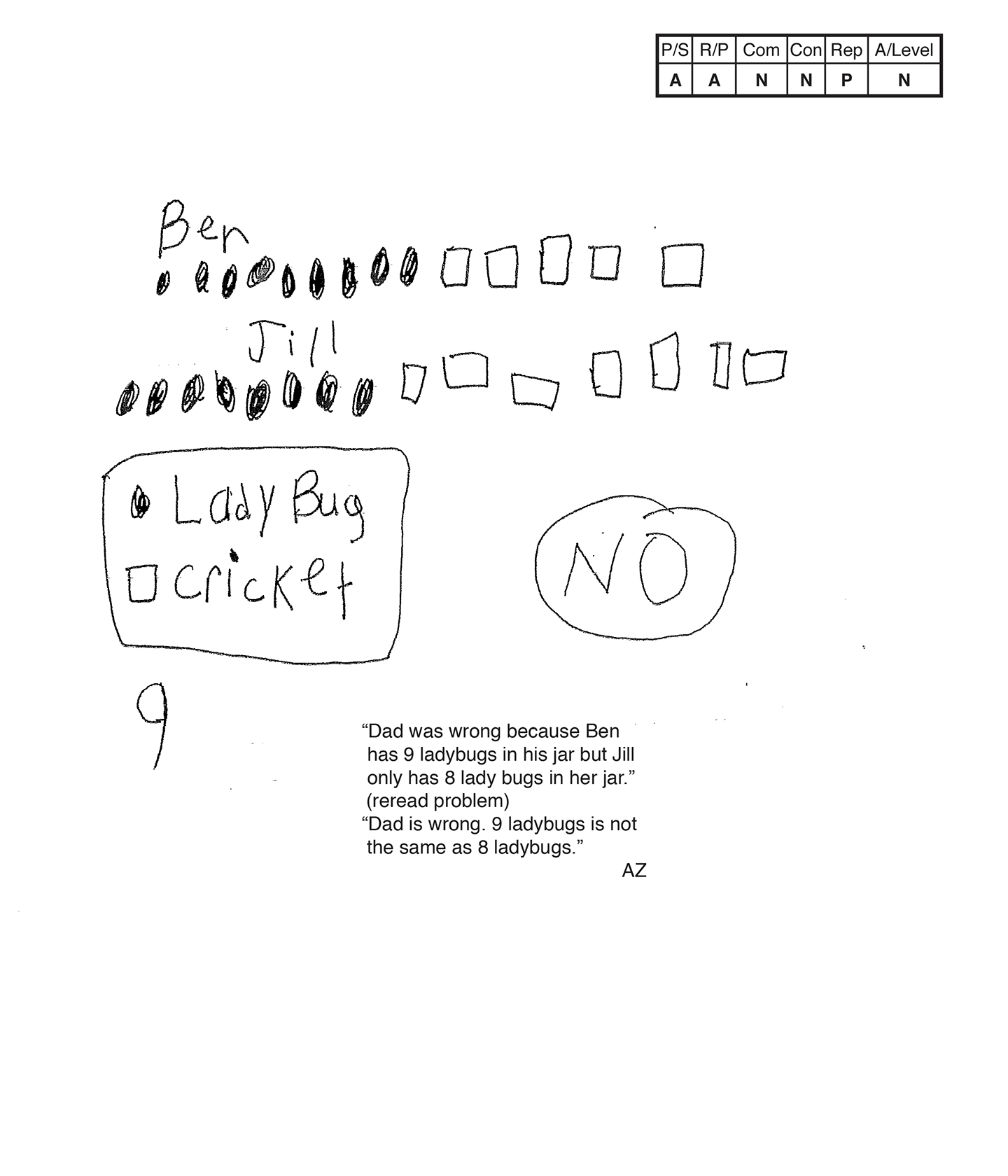This student does not meet the standard.

### Scoring Rationale

#### Apprentice

The student's strategy of diagramming the ladybugs and crickets Ben and Jill have in their jars works to solve part of the task. The student's answer, "NO," is not correct because it is based on comparing nine ladybugs to eight ladybugs and not the total number of insects in each jar.

#### Apprentice

The student shows some correct reasoning of the underlying concepts of the task. The student diagrams the correct number of ladybugs and crickets in each jar. The student does not demonstrate understanding that a comparison of the total of insects per jar has to be considered.

#### Novice

The student does not use any mathematical language to communicate their reasoning and proof.

#### Novice

The student does not make a mathematically relevant observation about their solution.

#### Practitioner

The student's diagram is appropriate to the task and accurate. A key defines the ladybugs and crickets and the total numbers of insects in each jar is correct.

#### Novice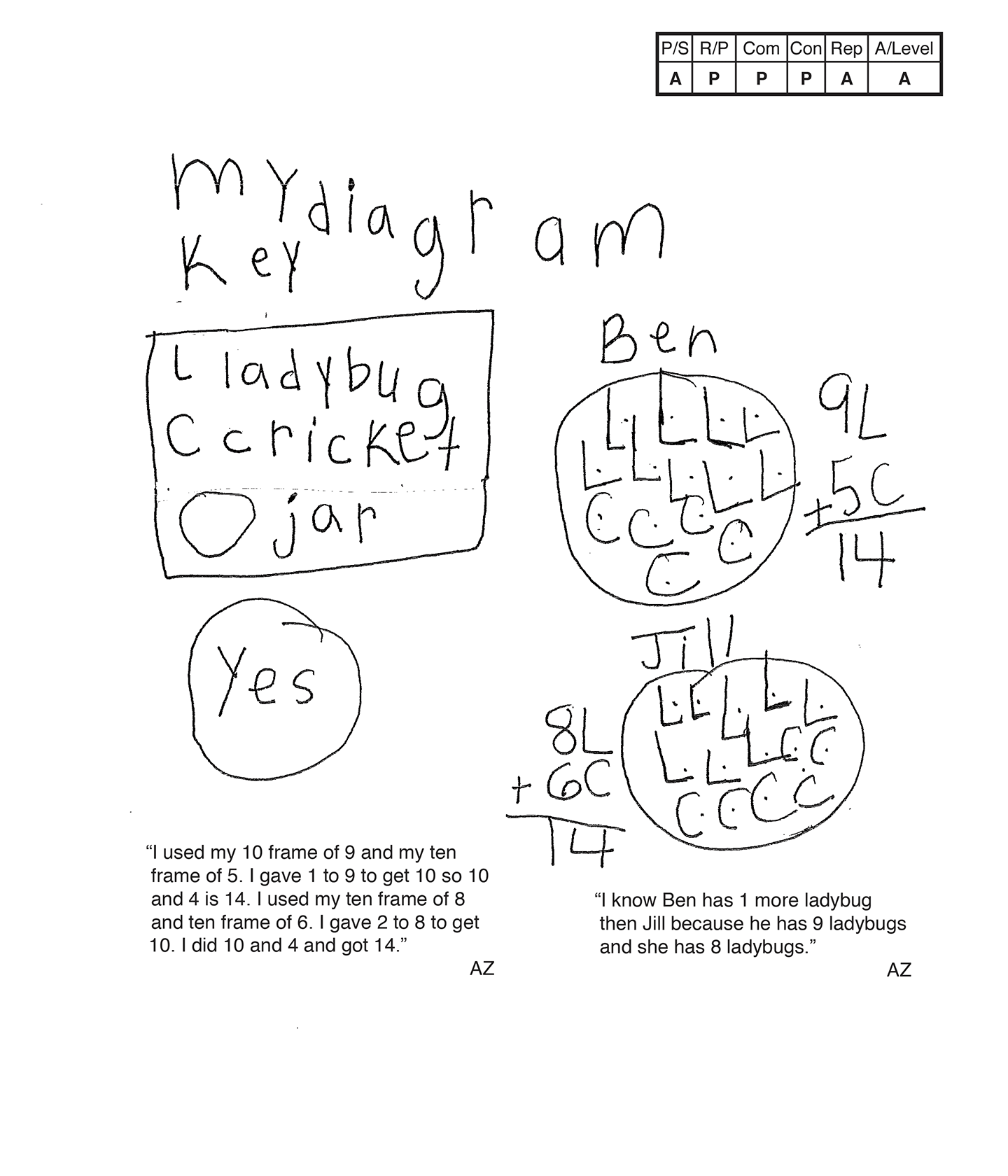This student does not meet the standard.

### Scoring Rationale

#### Apprentice

The student's strategy of diagramming the ladybugs and crickets Ben and Jill have in their jars would work to solve the task. The student omits one cricket from Jill's jar, which leads to an incorrect answer, "Yes."

#### Practitioner

The student shows correct reasoning of the underlying concepts of the task. The student understands that a total number of insects in each jar needs to be determined and the two totals compared to determine if Dad is correct. Omitting one cricket from Jill's jar is considered a careless error and not a flaw in the student's reasoning.

#### Practitioner

The student correctly uses the mathematical terms diagram, key, more than.

#### Practitioner

The student makes the mathematically relevant observation, "I know Ben has one more ladybug than Jill because he has nine ladybugs and she has eight ladybugs."

#### Apprentice

The student's diagram is appropriate to the task but is not accurate. The seventh cricket is missing from Jill's jar. A key defines the ladybugs, crickets, and jars.

#### Apprentice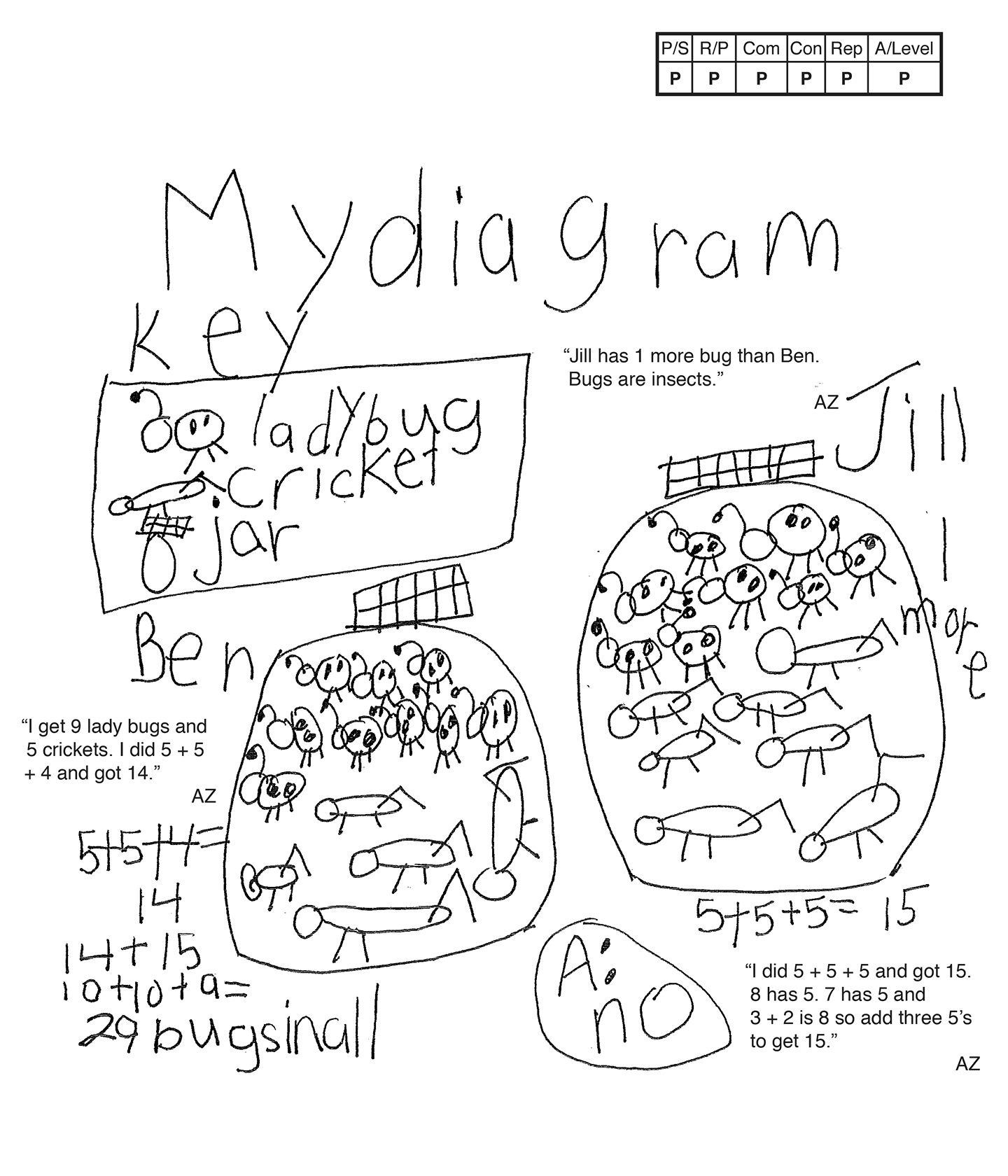This student meets the standard.

### Scoring Rationale

#### Practitioner

The student's strategy of diagramming the ladybugs and crickets Ben and Jill have in their jars, finding the total number of insects per jar, and comparing the totals to see if Dad is correct, works to solve the task. The student's answer, "A: no," is correct.

#### Practitioner

The student shows correct reasoning of the underlying concepts of the task. The student understands that a total number of insects in each jar needs to be determined and the two totals compared to determine if Dad is correct.

#### Practitioner

The student correctly uses the mathematical terms diagram, key, more than.

#### Practitioner

The student makes the mathematically relevant observations, "Jill has one more bug than Ben," and, "14 + 15, 10 + 10 + 9 = 29 bugs in all."

#### Practitioner

The student's diagram is appropriate to the task and accurate. A key defines the ladybugs, crickets, and jars. Each jar contains the correct number of each insect.

#### Practitioner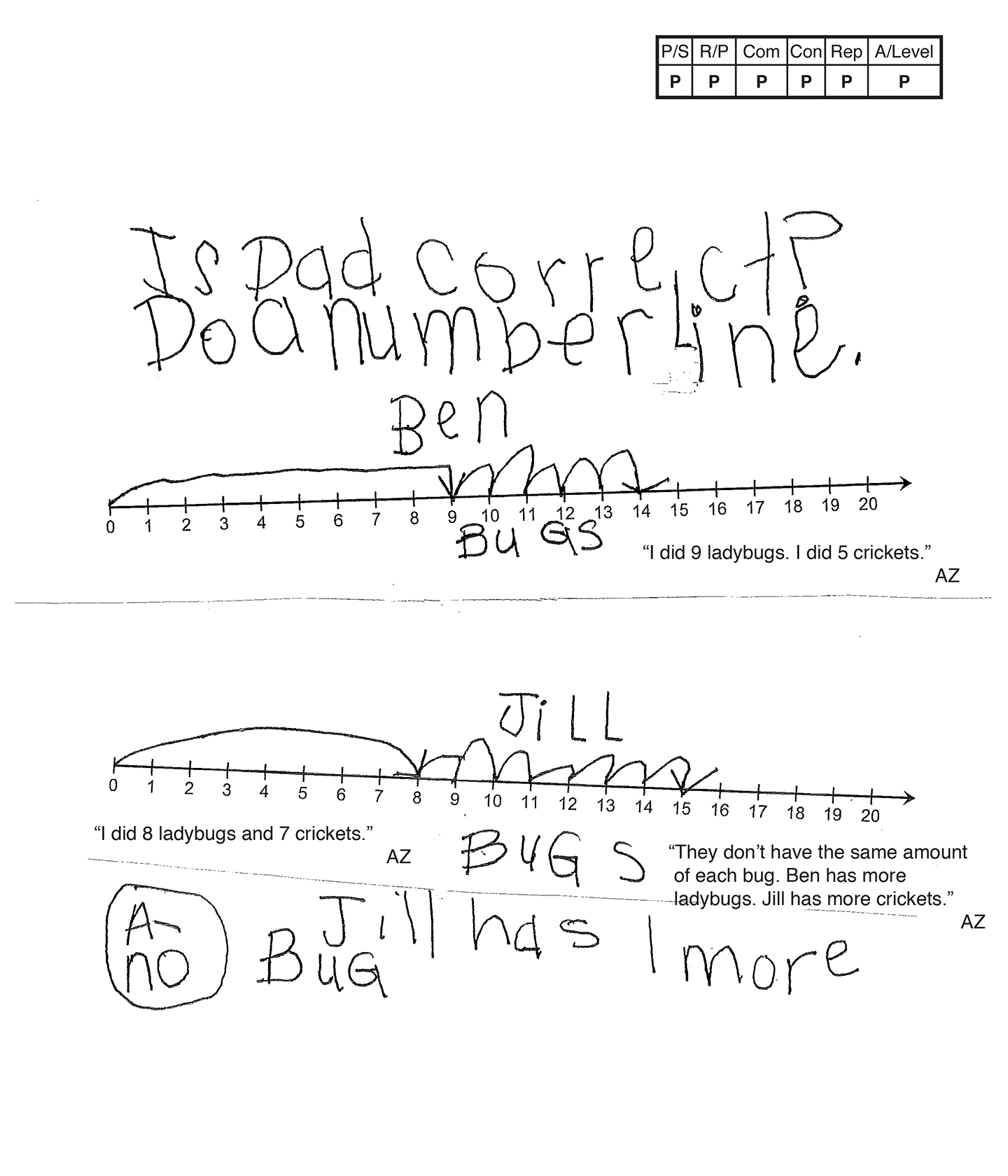This student meets the standard.

### Scoring Rationale

#### Practitioner

The student's strategy of using a number line to indicate the ladybugs and crickets Ben and Jill have in their jars, finding the total number of insects per jar, and comparing the totals to see if Dad is correct, works to solve the task. The student's answer, "A-no," is correct.

#### Practitioner

The student shows correct reasoning of the underlying concepts of the task. The student understands that a total number of insects in each jar needs to be determined and the two totals compared to determine if Dad is correct.

#### Practitioner

The student correctly uses the mathematical term amount from the task. The student also correctly uses the terms number line, more.

#### Practitioner

The student makes the mathematically relevant observations, "They don't have the same amount of each bug," "Ben has more ladybugs," "Jill has more crickets," and, "Jill has 1 more bug."

#### Practitioner

The student's number line is appropriate to the task and accurate. All necessary labels are indicated and the student's "jumps" are correct.

#### Practitioner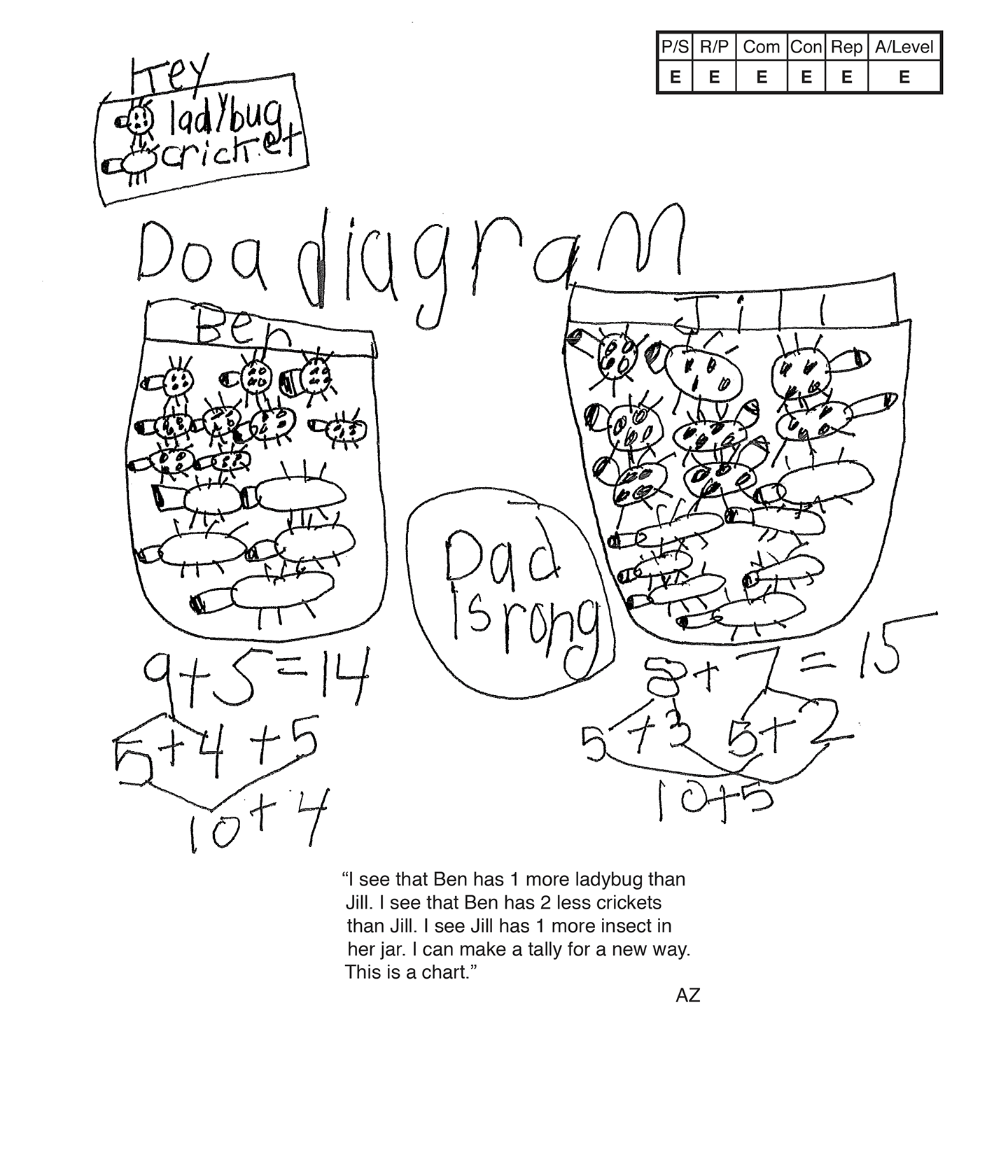,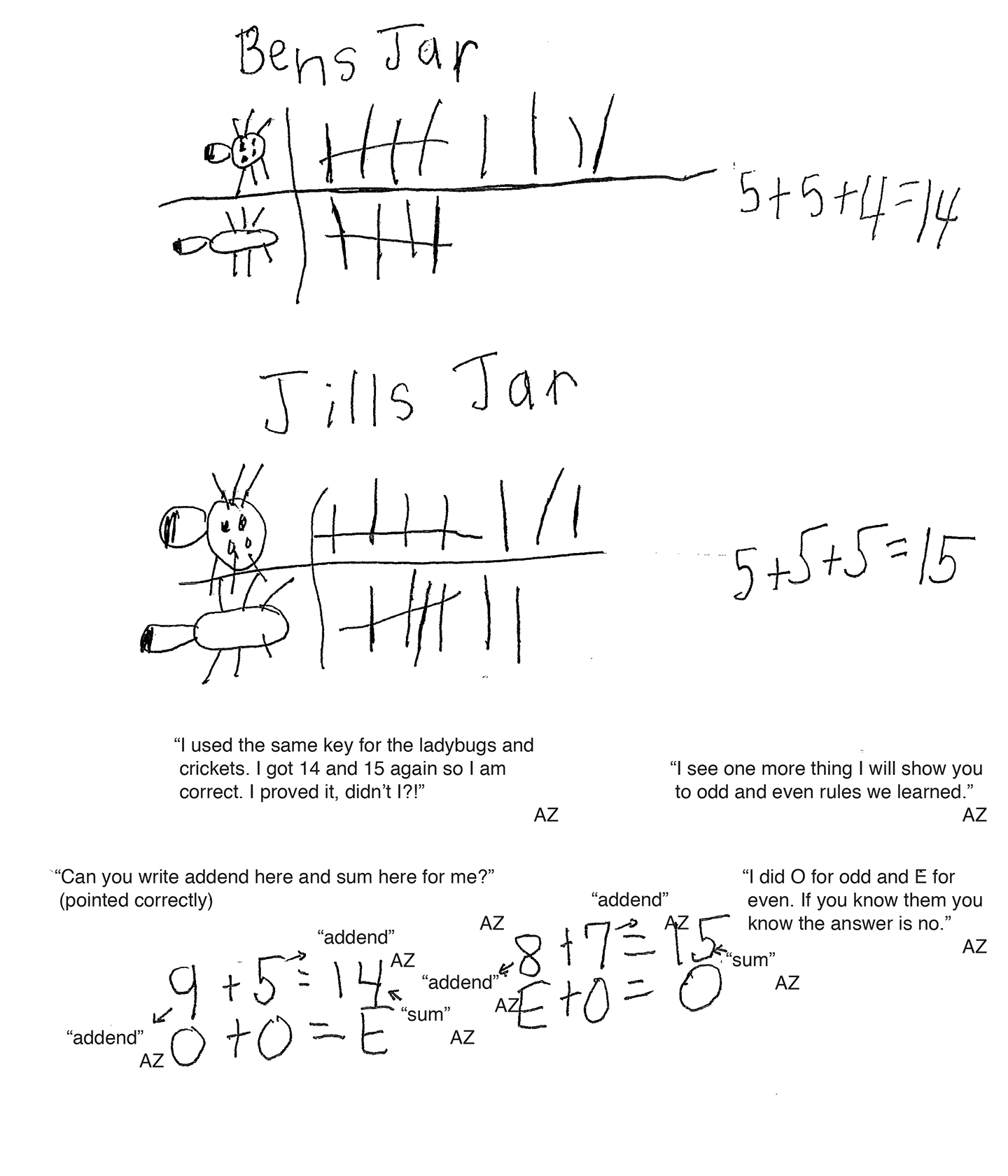This student exceeds the standard.

### Scoring Rationale

#### Expert

The student's strategy of using a diagram to indicate the ladybugs and crickets Ben and Jill have in their jars, finding the total number of insects per jar, and comparing the totals to see if Dad is correct, works to solve the task. The student's answer, "Dad is rong," is correct. The student verifies that their answer is correct by using a new strategy of a tally chart. The student also applies the rules for addition with even and odd numbers.

#### Expert

The student shows correct reasoning of the underlying concepts of the task. The student understands that a total number of insects in each jar needs to be determined and the two totals compared to determine if Dad is correct. The student uses a tally chart to verify that their answer is correct and also applies the addition rules using odd and even numbers.

#### Expert

The student correctly uses the mathematical terms key, diagram, more than, less than, more, tally, chart, odd, even, rules, addend, sum. The student correctly uses the notation "O + O = E" and "E + O = O" and defines the O (odd) and E (even) in the scribing.

#### Expert

The student makes the mathematically relevant Practitioner observations, "I see that Ben has one more ladybug than Jill," "I see that Ben has two less crickets than Jill," and, "I see Jill has one more insect in her jar." The student makes the Expert connection by using a tally chart to verify that the student's answer is correct. The student states, "I used the same key for the ladybug and crickets. I got fourteen and fifteen again so I am correct. I proved it didn't I!" The student uses the odd and even rules. 9 + 5 = 14, O + O = E, 8 + 7 = 15, E + O = O. The student states, "I did O for Odd and E for Even. If you know them you know the answer is no."

#### Expert

The student's diagram is appropriate and accurate. All necessary labels are included. The student's tally chart is appropriate and accurate. All necessary labels are included and supported by the student's text. The student compares their tally chart to their diagram to verify that their answer is correct.